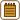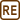## Adiabatic Compression in a Pump

### Task number: 2172

Air at a temperature of 20 °C is enclosed in a circular cylinder of a pump by a piston. The piston is located at a distance of 50 cm from the bottom of the cylinder. How will the temperature and pressure of this air change, if we move the piston swiftly with no friction by 20 cm in the direction of the bottom of the cylinder?

• #### Hint: Which process is taking place?

Rapid compression is a very quick process, so we can assume that there is no heat transfer between the air in the pump and its surroundings.

In such case we say that it is an adiabatic process, which can be described by Poisson's equation.

• #### Hint: What about the unknown volume?

Although neither initial, nor final volume of the gas is known, if we express the final pressure from Poisson's equation, we will find out that we need only the ratio of the volumes.

The ratio of the initial and final volume is the same as the ratio of the length of the pump cylinder at the beginning and at the end of compression.

• #### Notation

 l = 50 cm initial distance of the piston from the bottom d = 20 cm displacement of the piston p = ? pressure of the air after compression t = ? temperature of the air after compression

Other values:

 p0 = 1.01325·105 Pa normal atmospheric pressure t0 = 20 °C ⇒ T0 = 293 K initial temperature of the air κ = 1.39 Poisson's ratio for the air

(the air consists of gases with diatomic molecules mostly, so we could also use the value κ = 1.4)

• #### Analysis

We will consider the air in the pump to be ideal gas. If the compression truly happens rapidly , there will be no heat transfer between the air and surroundings and we can consider this process to be adiabatic. We express the final pressure of the gas from the Poisson's equation for adiabatic process. The temperature after compression can be obtained from the equation of state for ideal gas.

In both equations though, we have the initial and final volume of the air, which we don't know. Yet, it appears as the ratio of the two volumes, and we can express this ratio from the height of the cylinder and the displacement of the piston during compression.

• #### Solution

First, we express the ratio of the initial volume V0 and the final volume V of the air in the pump. Let's denote the unknown area of the base of the cylinder by A. Then the following holds true:

$\frac{V_0}{V}\,=\,\frac{lA}{\left(l-d\right)A}\,=\,\frac{l}{\left(l-d\right)}\,.$

Quick compression can be considered to be an adiabatic process, and Poisson's equation can be applied to the initial and final pressure and volume:

$p_0V_0^{\kappa}\,=\,pV^{\kappa}.$

Therefrom we express the unknown pressure p and substitute the ratio of the volumes:

$p\,=\,\frac{p_0V_0^{\kappa}}{V^{\kappa}}\,=\,p_0\frac{V_0^{\kappa}}{V^{\kappa}}\,=\,p_0\left(\frac{V_0}{V}\right)^{\kappa}\,=\,p_0\left(\frac{l}{l-d}\right)^{\kappa}.$

Final temperature of the gas can be determined from the equation of state for ideal gas:

$\frac{pV}{T}\,=\,\frac{p_0V_0}{T_0}\,.$

We express the unknown temperature of the gas T and substitute the previous relations:

$T\,=\,\frac{T_0pV}{p_0V_0}\,=\,T_0\,\frac{p}{p_0}\,\frac{V}{V_0}\,=\,T_0\frac{p_0\left(\frac{l}{l-d}\right)^{\kappa}}{p_0}\,\frac{l-d}{l}\,=\,T_0\left(\frac{l}{l-d}\right)^{\kappa -1}.$

After substitution for the given values:

$p\,=\,1{.}01325\cdot{10^5}\cdot\left(\frac{50}{50-20}\right)^{1{.}39}\,\mathrm{Pa}\,\dot{=}\,2{.}1\cdot{ 10^5}\,\mathrm{Pa}$ $T\,=\,293\cdot\left(\frac{50}{50-20}\right)^{1{.}39-1}\,\mathrm{K}\,\dot{=}\,358\,\mathrm{K}\,\Rightarrow\,t\,=\,85\,{}^{\circ}\mathrm{C}.$
• #### Answer

After the rapid compression the pressure of the air will increase approximately twice, i.e. to the value of 2.1·105 Pa, and the temperature will increase roughly to 85 °C.